## End Behavior of Power Functions

### Learning Outcomes

• Identify a power function.
• Describe the end behavior of a power function given its equation or graph.Three birds on a cliff with the sun rising in the background. Functions discussed in this module can be used to model populations of various animals, including birds. (credit: Jason Bay, Flickr)

Suppose a certain species of bird thrives on a small island. Its population over the last few years is shown below.

 Year 2009 2010 2011 2012 2013 Bird Population 800 897 992 1,083 1,169

The population can be estimated using the function $P\left(t\right)=-0.3{t}^{3}+97t+800$, where $P\left(t\right)$ represents the bird population on the island t years after 2009. We can use this model to estimate the maximum bird population and when it will occur. We can also use this model to predict when the bird population will disappear from the island.

### Identifying Power Functions

In order to better understand the bird problem, we need to understand a specific type of function. A power function is a function with a single term that is the product of a real number, coefficient, and variable raised to a fixed real number power. Keep in mind a number that multiplies a variable raised to an exponent is known as a coefficient.

As an example, consider functions for area or volume. The function for the area of a circle with radius $r$ is:

$A\left(r\right)=\pi {r}^{2}$

and the function for the volume of a sphere with radius r is:

$V\left(r\right)=\frac{4}{3}\pi {r}^{3}$

Both of these are examples of power functions because they consist of a coefficient, $\pi$ or $\frac{4}{3}\pi$, multiplied by a variable r raised to a power.

### A General Note: Power FunctionS

A power function is a function that can be represented in the form

$f\left(x\right)=a{x}^{n}$

where a and n are real numbers and a is known as the coefficient.

### Q & A

Is $f\left(x\right)={2}^{x}$ a power function?

No. A power function contains a variable base raised to a fixed power. This function has a constant base raised to a variable power. This is called an exponential function, not a power function.

### Example: Identifying Power Functions

Which of the following functions are power functions?

$\begin{array}{c}f\left(x\right)=1\hfill & \text{Constant function}\hfill \\ f\left(x\right)=x\hfill & \text{Identify function}\hfill \\ f\left(x\right)={x}^{2}\hfill & \text{Quadratic}\text{ }\text{ function}\hfill \\ f\left(x\right)={x}^{3}\hfill & \text{Cubic function}\hfill \\ f\left(x\right)=\frac{1}{x} \hfill & \text{Reciprocal function}\hfill \\ f\left(x\right)=\frac{1}{{x}^{2}}\hfill & \text{Reciprocal squared function}\hfill \\ f\left(x\right)=\sqrt{x}\hfill & \text{Square root function}\hfill \\ f\left(x\right)=\sqrt{x}\hfill & \text{Cube root function}\hfill \end{array}$

### Try It

Which functions are power functions?

$\begin{array}{c}f\left(x\right)=2{x}^{2}\cdot 4{x}^{3}\hfill \\ g\left(x\right)=-{x}^{5}+5{x}^{3}-4x\hfill \\ h\left(x\right)=\frac{2{x}^{5}-1}{3{x}^{2}+4}\hfill \end{array}$

### Identifying End Behavior of Power Functions

The graph below shows the graphs of $f\left(x\right)={x}^{2},g\left(x\right)={x}^{4}$, $h\left(x\right)={x}^{6}$, $k(x)=x^{8}$, and $p(x)=x^{10}$ which are all power functions with even, whole-number powers. Notice that these graphs have similar shapes, very much like that of the quadratic function. However, as the power increases, the graphs flatten somewhat near the origin and become steeper away from the origin.

To describe the behavior as numbers become larger and larger, we use the idea of infinity. We use the symbol $\infty$ for positive infinity and $-\infty$ for negative infinity. When we say that “x approaches infinity,” which can be symbolically written as $x\to \infty$, we are describing a behavior; we are saying that x is increasing without bound.

With even-powered power functions, as the input increases or decreases without bound, the output values become very large, positive numbers. Equivalently, we could describe this behavior by saying that as $x$ approaches positive or negative infinity, the $f\left(x\right)$ values increase without bound. In symbolic form, we could write

$\text{as }x\to \pm \infty , f\left(x\right)\to \infty$

The graph below shows $f\left(x\right)={x}^{3},g\left(x\right)={x}^{5},h\left(x\right)={x}^{7},k\left(x\right)={x}^{9},\text{and }p\left(x\right)={x}^{11}$, which are all power functions with odd, whole-number powers. Notice that these graphs look similar to the cubic function. As the power increases, the graphs flatten near the origin and become steeper away from the origin.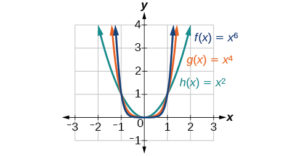These examples illustrate that functions of the form $f\left(x\right)={x}^{n}$ reveal symmetry of one kind or another. First, in the even-powered power functions, we see that even functions of the form $f\left(x\right)={x}^{n}\text{, }n\text{ even,}$ are symmetric about the y-axis. In the odd-powered power functions, we see that odd functions of the form $f\left(x\right)={x}^{n}\text{, }n\text{ odd,}$ are symmetric about the origin.

For these odd power functions, as x approaches negative infinity, $f\left(x\right)$ decreases without bound. As x approaches positive infinity, $f\left(x\right)$ increases without bound. In symbolic form we write

$\begin{array}{c}\text{as } x\to -\infty , f\left(x\right)\to -\infty \\ \text{as } x\to \infty , f\left(x\right)\to \infty \end{array}$

The behavior of the graph of a function as the input values get very small ( $x\to -\infty$ ) and get very large ( $x\to \infty$ ) is referred to as the end behavior of the function. We can use words or symbols to describe end behavior.

The table below shows the end behavior of power functions of the form $f\left(x\right)=a{x}^{n}$ where $n$ is a non-negative integer depending on the power and the constant.

Even power Odd power
Positive constant

a > 0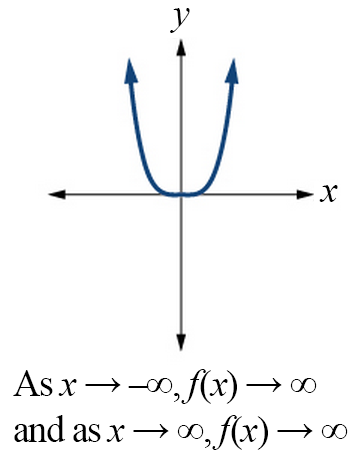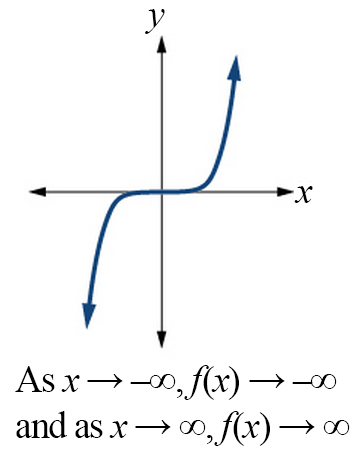Negative constant

a < 0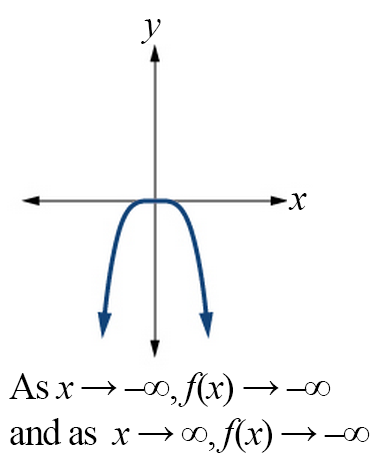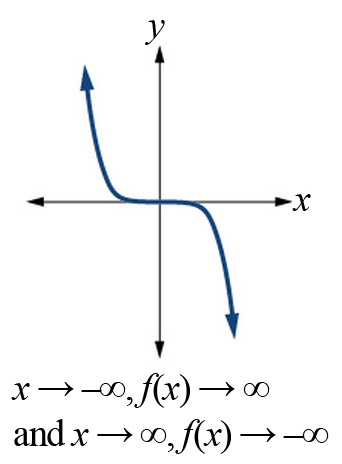### How To: Given a power function $f\left(x\right)=a{x}^{n}$ where $n$ is a non-negative integer, identify the end behavior.

1. Determine whether the power is even or odd.
2. Determine whether the constant is positive or negative.
3. Use the above graphs to identify the end behavior.

### Example: Identifying the End Behavior of a Power Function

Describe the end behavior of the graph of $f\left(x\right)={x}^{8}$.

### Example: Identifying the End Behavior of a Power Function

Describe the end behavior of the graph of $f\left(x\right)=-{x}^{9}$.

### Try It

Describe in words and symbols the end behavior of $f\left(x\right)=-5{x}^{4}$.

## Contribute!

Did you have an idea for improving this content? We’d love your input.